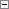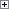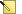After you select an identification number and name for a field, you have to select an appropriate data type. Each of the different field types is designed to hold a specific kind of information, such as text, numbers, or dates. Each field type has a specific size.Note
Sizes are rounded up to the nearest 4 bytes.

Fields in a record can be of the following types.

Data type Description Size

BigInteger Data Type

A 64-bit integer.

8 bytes

BLOB Data Type

Binary Large Object. Used to store bitmaps and memos. Notice that the BLOB is not stored in the record, but in the BLOB area of the table.

The size of the corresponding SQL data type, IMAGE, is the number of bytes in the field’s value. (A)(B)

8 bytes in the record + size of BLOB data (maximum 2 GB)

Boolean Data Type

Assumes the values TRUE or FALSE. When formatted, a Boolean field is shown as "Yes" or "No". The size of the corresponding SQL data type, TINYINT, is 1 byte. (A)(B)

4 bytes

Code Data Type

An alphanumeric string, which is right-justified if the contents are numbers only. If letters or blanks occur among the numbers, the contents are left-aligned. All letters are converted to uppercase upon entry.

The field must be defined to be between 1 and 250 characters. The number of bytes used by a Code field equals 1 byte + ((number of characters + 1) * 2). The initial byte is for the leading indicator, which specifies the length of a Code value that consists only of integers. The additional character is used for the string terminating character, which is '0' in Unicode. The size of a Unicode character is 2 bytes. Therefore, you multiply the number of characters by two to get the bytes.

For example, if a Code value is 6 characters, then the number of bytes used is the following:

1 + ((6+1)*2) = 15, rounded to the nearest 4 bytes = 16 bytes used.

You can use the SQL Data Type Property to indicate whether Code fields can contain integers or text strings.

For more information about the sorting of numeric values in code fields, see Sorting Numeric Values.

1 byte + ((Number of characters + 1 character) * 2 bytes per character)

Date Data Type

A date value in the range from January 1, 0000 to December 31, 9999. An undefined date is expressed as 0. All dates have a corresponding closing date. The system considers the closing date for a given date as a period that follows the given date but comes before the next normal date; that is, a closing date is sorted immediately after the corresponding normal date but before the next normal date.

The size of the corresponding SQL data type, DATETIME, is 8 bytes. (A)(B)

4 bytes

DateFormula Data Type

Used to verify the date entered by the user. The syntax is for example:Copy Code
30D (=30 days)
CM+1M (=current month plus one month)
D15 (=on the 15th of each month)

32 bytes

DateTime Data Type

Represents a point in time as a combined date and time. The datetime is stored in the database as Coordinated Universal Time (UTC) and is always displayed as local time in Microsoft Dynamics NAV.

Local time is determined by the time zone regional settings that are used by your computer.

You must always enter datetimes as local time. When you enter a datetime as local time, it is converted to UTC using the current settings for the time zone and daylight saving time.

The DateTime data type does not support closing dates.

Stored as two 4 byte integers

Decimal Data Type

A decimal number between -10^63 and 10^63. The exponent ranges from -63 to 63. Decimal numbers are held in memory with 18 significant digits. The representation of a decimal number is a Binary Coded Decimal (BCD). The size of the corresponding SQL data type, DECIMAL(38,20), is 17 bytes. We recommend that you construct decimals that operate on numbers in the range of +/- 999,999,999,999,999.99. You can construct larger numbers in some cases, but overflow, truncation or loss of precision can occur.

(A)(B)

12 bytes

Duration Data Type

Represents the difference between two points in time, in milliseconds. This value can be negative.

8 bytes

GUID Data Type

Globally unique identifier (GUID).

16 bytes

Integer Data Type

Denotes an integer between -2,147,483,647 and 2,147,483,647. The size of the corresponding SQL data type, INTEGER, is 4 bytes. (A)(B)

4 bytes

Option Data Type

An option field is defined by using an option string, which is a comma-separated list of strings that represent each valid value of the field. This string is used when a field of type Option is formatted and its value is converted into a string.

For example, the Option field "Color" is defined by using the option string "Red,Green,Blue". Valid values of the field are then 0, 1, and 2, with 0 representing "Red" and so on. When the "Color" field is formatted, 0 is converted into the string "Red", 1 into "Green", and 2 into "Blue".

The size of the corresponding SQL data type, INTEGER, is 4 bytes. (A)(B)

4 bytes

RecordID Data Type

Unique record identifier.

TableFilter Data Type

This data type is used to apply a filter to another table.

Currently, this can only be used to apply security filters from the Permission table.

Text Data Type

Any alphanumeric string. The field must be defined to be between 1 and 250 characters. The number of bytes used by a text field equals (number of characters + 1) * 2. The additional character is used for the string terminating character, which is '0' in Unicode. The size of a Unicode character is 2 bytes. Therefore, you multiply the number of characters by two to get the size.

For example, if a Text value is 6 characters, then the number of bytes used is the following:

(6+1)*2 = 14 bytes, rounded up to the nearest 4 bytes = 16 bytes used.

An empty text string has the length zero.

(Number of characters+ 1 character) * 2 bytes per character

Time Data Type

Any time in the range 00:00:00 to 23:59:59.999. A time field contains 1 plus the number of milliseconds since 00:00:00 o'clock, or 0 (zero), an undefined time. A time value is calculated in the following way:Copy Code
Time = 1 + (number of milliseconds since 00:00:00).

The size of the corresponding SQL data type, DATETIME, is 8 bytes. (A)(B)

A time field is stored as an integer (four bytes)

(A) The calculation of the size of a specific SQL Server record requires more than just summing the sizes of the field values. Refer to Microsoft SQL Server documentation for more information.

(B) This is the SQL Server data type that Microsoft Dynamics NAV uses when it creates the Microsoft Dynamics NAV data type. For more information, see Identifiers, Data Types, and Data Formats.

In addition to the fields discussed in this section, tables can include the following special types of fields that are used to retrieve data:

• FlowField
• FlowFilter

For more information, see FlowFields and FlowFilter Overview.

C/AL Functions